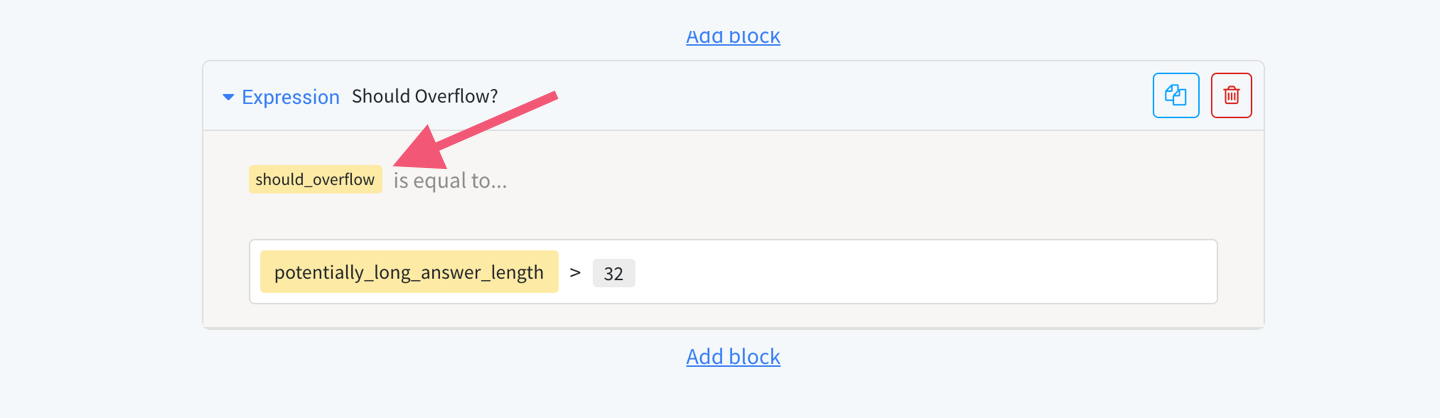# What to do if text doesn't fit into PDF field

September 9, 2021

If text doesn't fit into a PDF field, you have two options:

1. Force the end-user to write shorter text; or
2. Take the "overflow" text and automatically add it an addendum to the PDF.

### Option 1: Force the end-user to write shorter text

If you know how many characters can fit into a PDF field, you can evaluate whether the end-user's response is above or below that threshold. If it's above, you can force the end-user to shorten their response. This video explains how:

### Option 2: Reduce the font size of the text

To reduce the font size of the text in a particular field, click on that field and then reduce the point size of the font:

### Option 3: Take the "overflow" text and automatically add it an addendum to the PDF.

This solution allows you to conditionally create an addendum to your PDF that contains "overflow" text that can't fit on small PDF fields. If the end-user's input doesn't fit on the field, the sentence "Check addendum" is written in the PDF field and an addendum is automatically attached to the Block where you provide the original PDF.

#### How it works

Imagine you have a long text question that defines a variable, like so:

What you want to do is figure out whether this variable (potentially_long_answer) is greater than or equal to a certain number of characters (whatever number you believe the PDF's field can handle).

#### Step 1

Use an Expression Block (which allows you to create a variable with an expression editor) to create a variable that is equal to the number of characters in your long text question variable:

#### Step 2

Now that you have a variable that is equal to the number of characters in your long text variable, it's time to figure out whether that number is more than the PDF field can handle. This variable (which I am calling should_overflow) is what will determine whether or not we take the end-user's input and transport it to an addendum instead of adding to a too small PDF field.This expression is evaluating two numeric values. The first is the number of characters in my long text variable, the other numeric value is the limit of characters I believe the PDF field can handle

#### Step 3

Now you need to create the variable that is ultimately added to the PDF field. This variable will either equal the end user's input or the sentence "Check the addendum" depending on how many characters are in the end-user's input.

This is a perfect use-case for a Conditional Block:

Add answer_to_show_in_main_document to your PDF on the field that might be too small for the end-user's input.

#### Last step

Create the addendum. You can use Microsoft Word, but a simple addendum can also be easily built with the Afterpattern Word editor. On the addendum, simply add the long text question variable (in this example, the variable potentially_long_answer). You may also wish to add headers that explain what PDF field this answer corresponds to.

When you give the addendum to the end-user, make sure to use conditional logic to only provide the addendum if the addendum is necessary in the first place, like so: